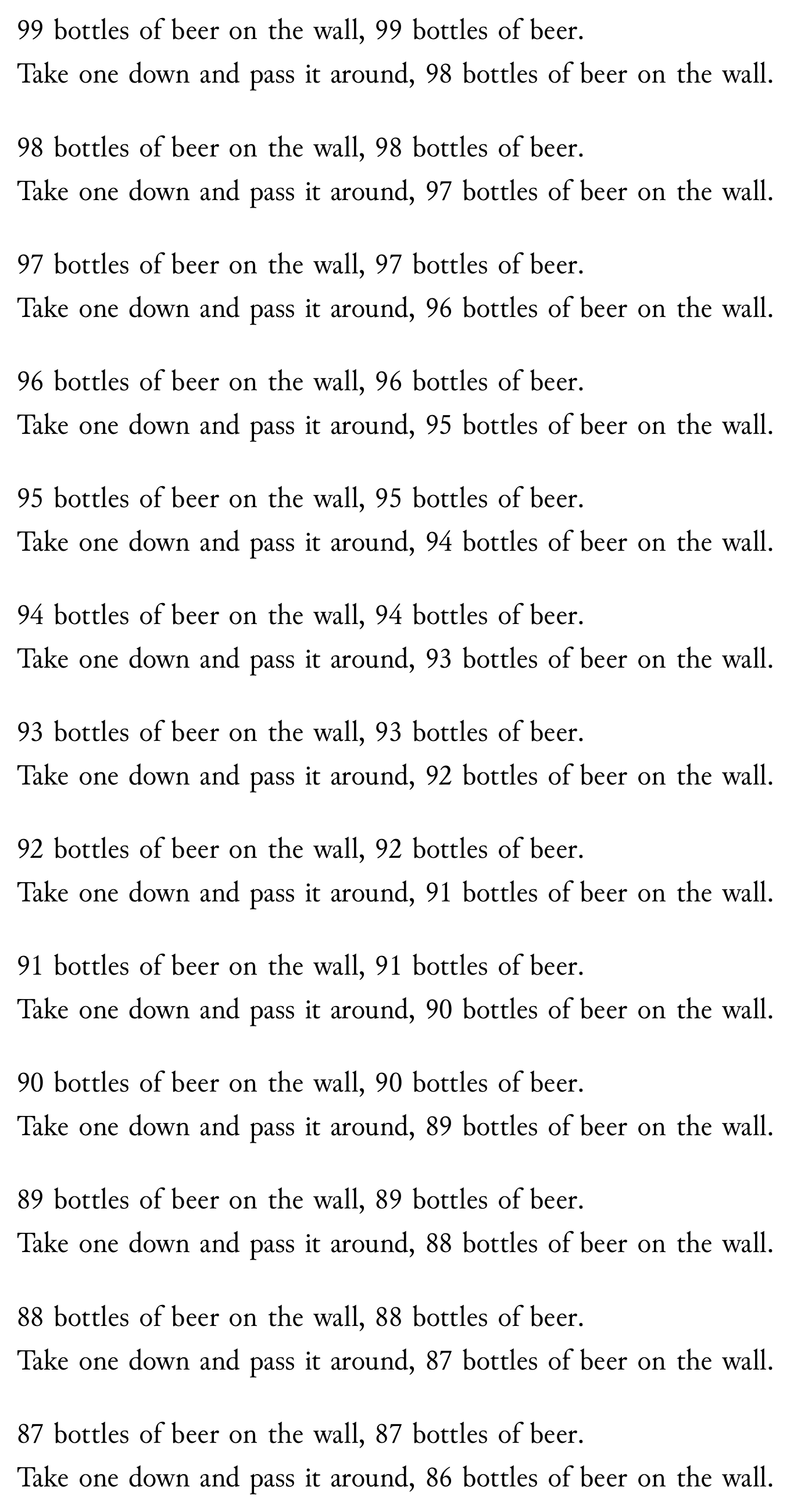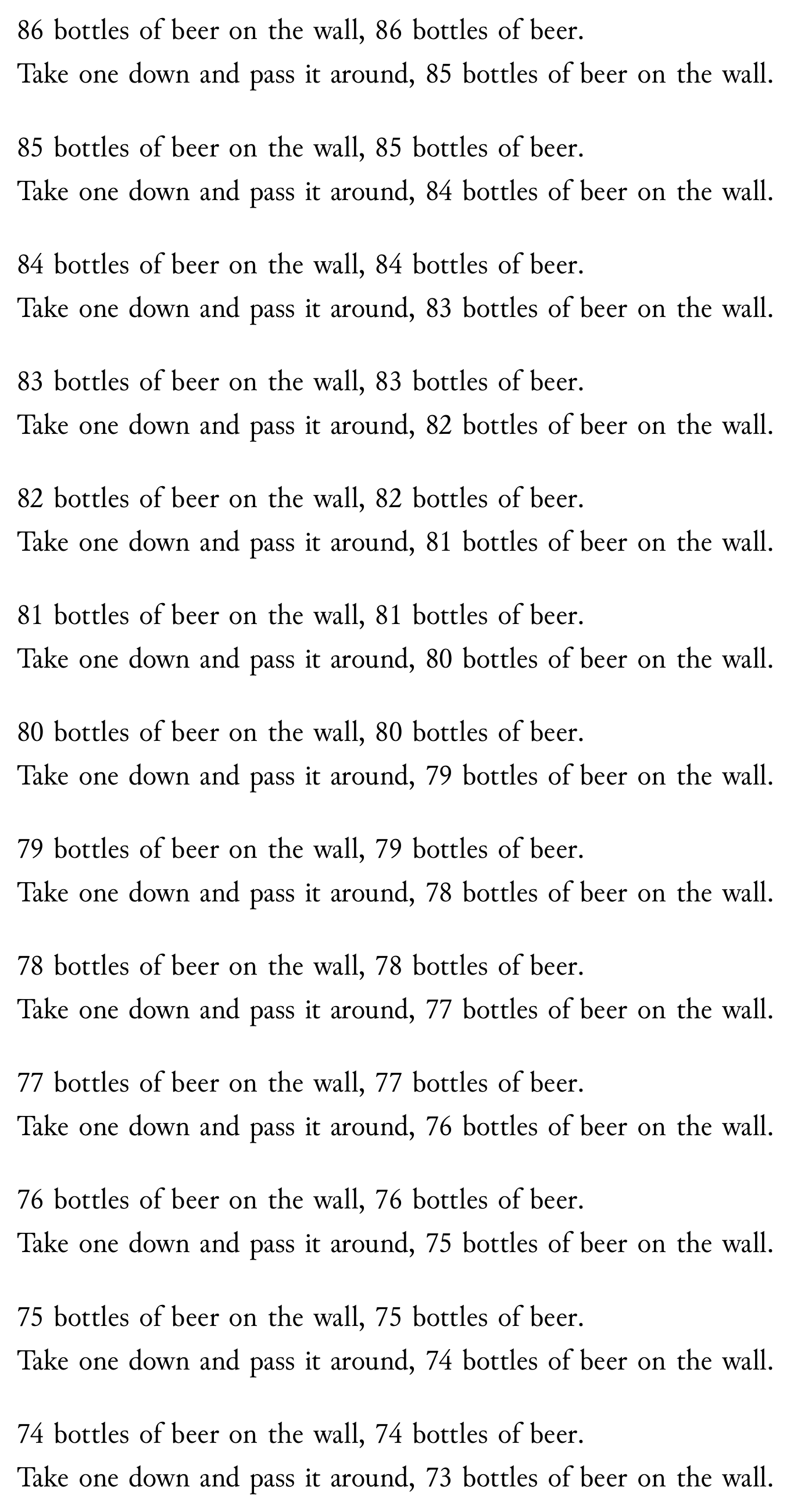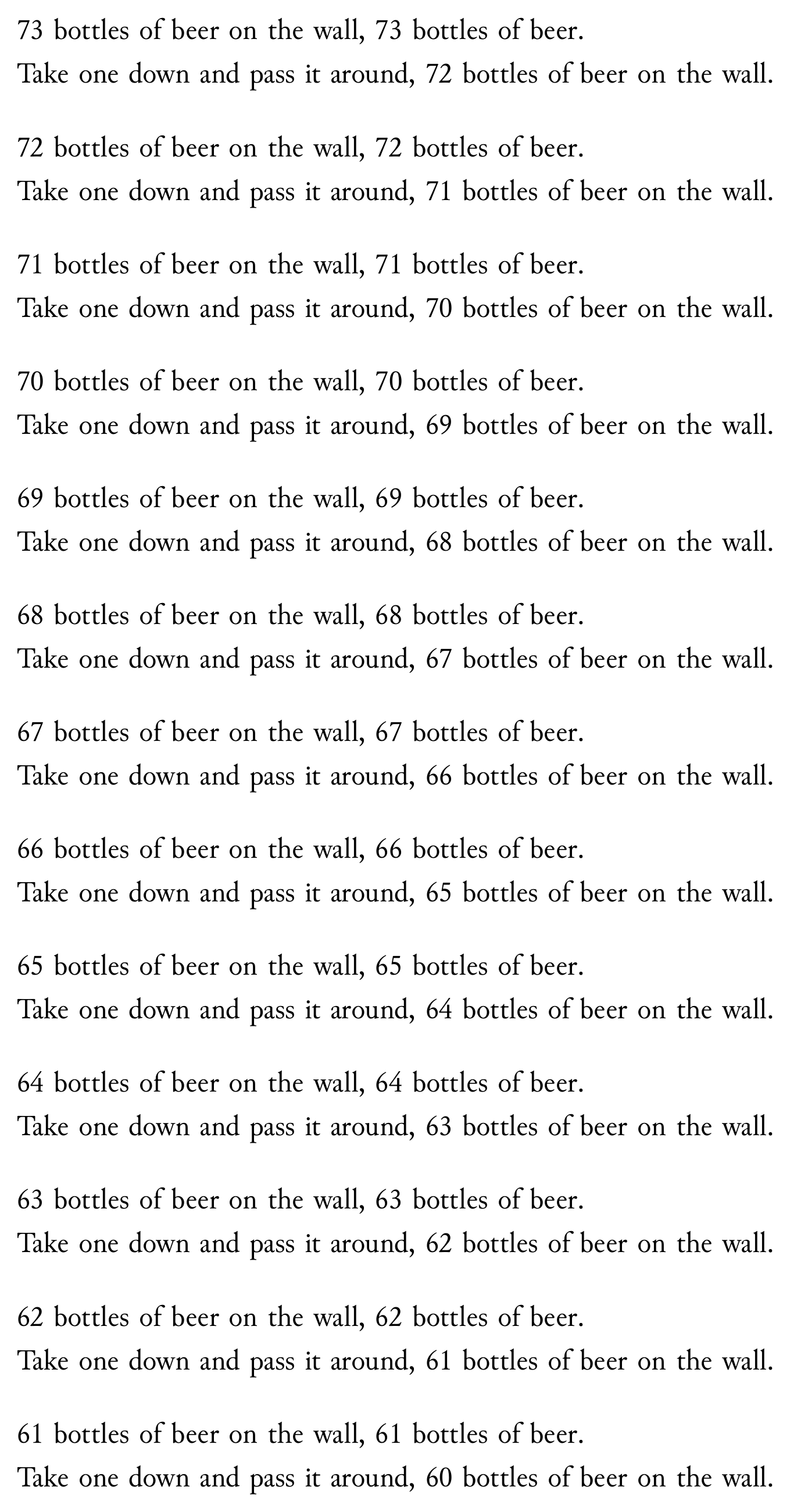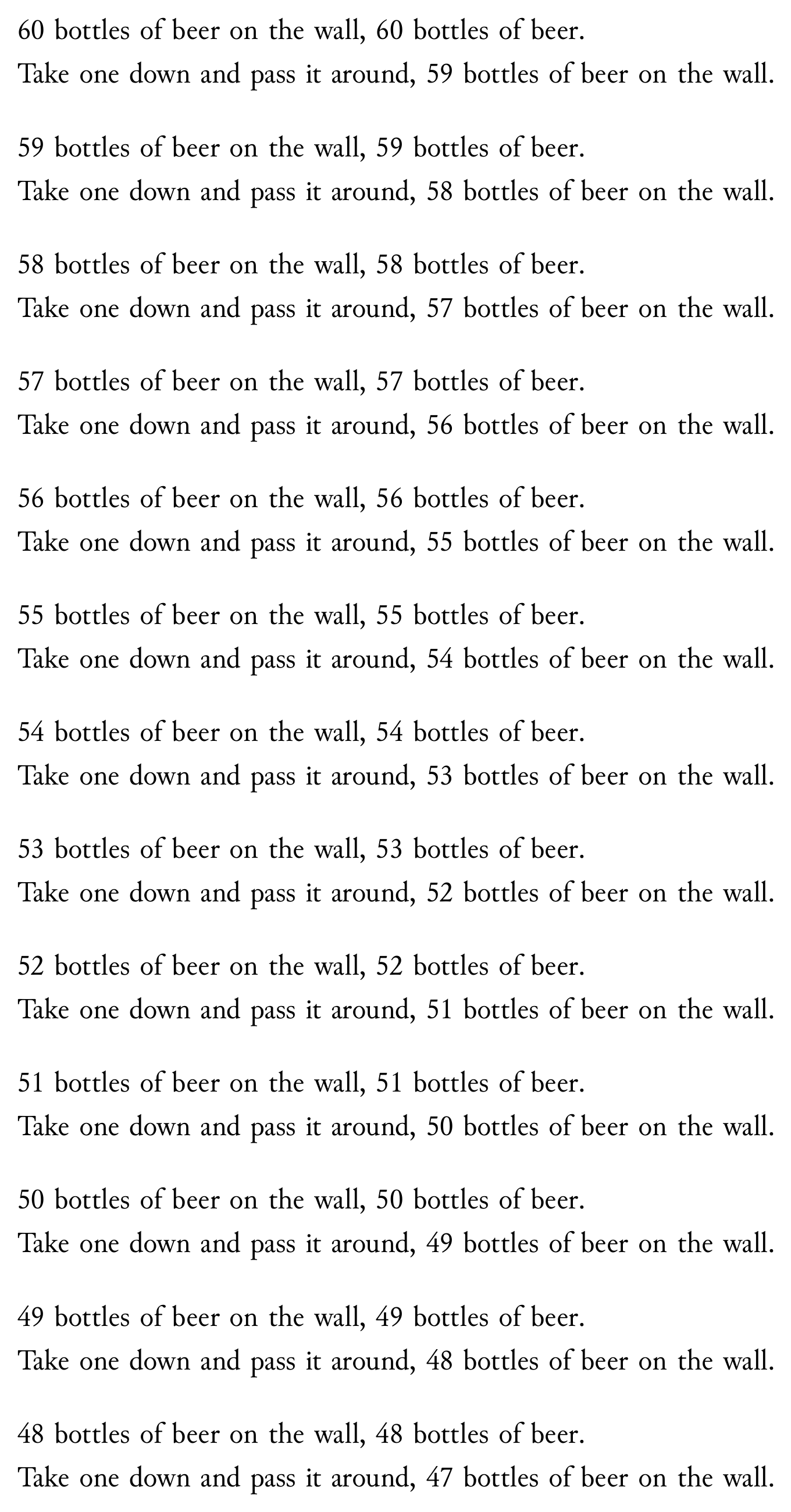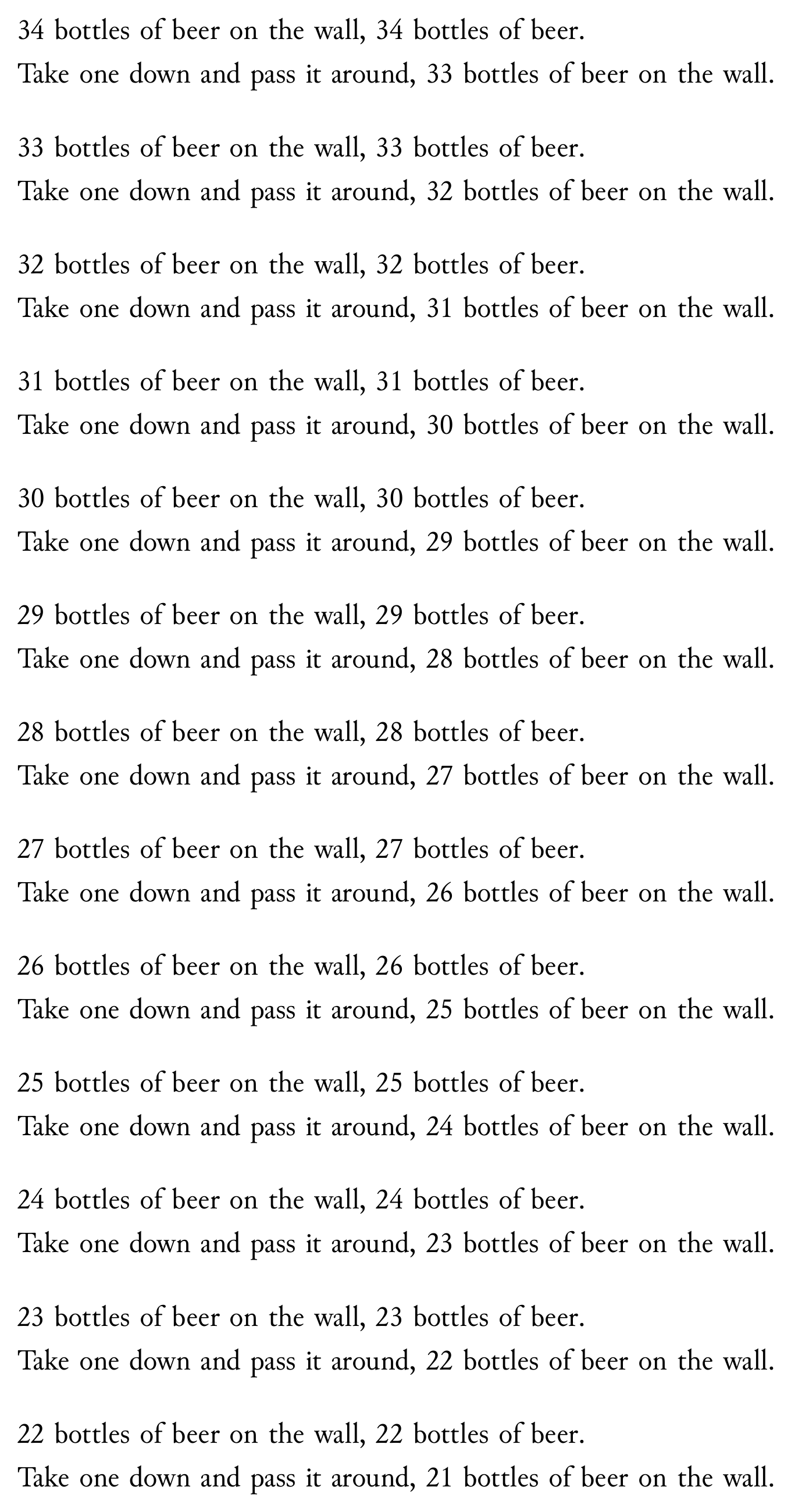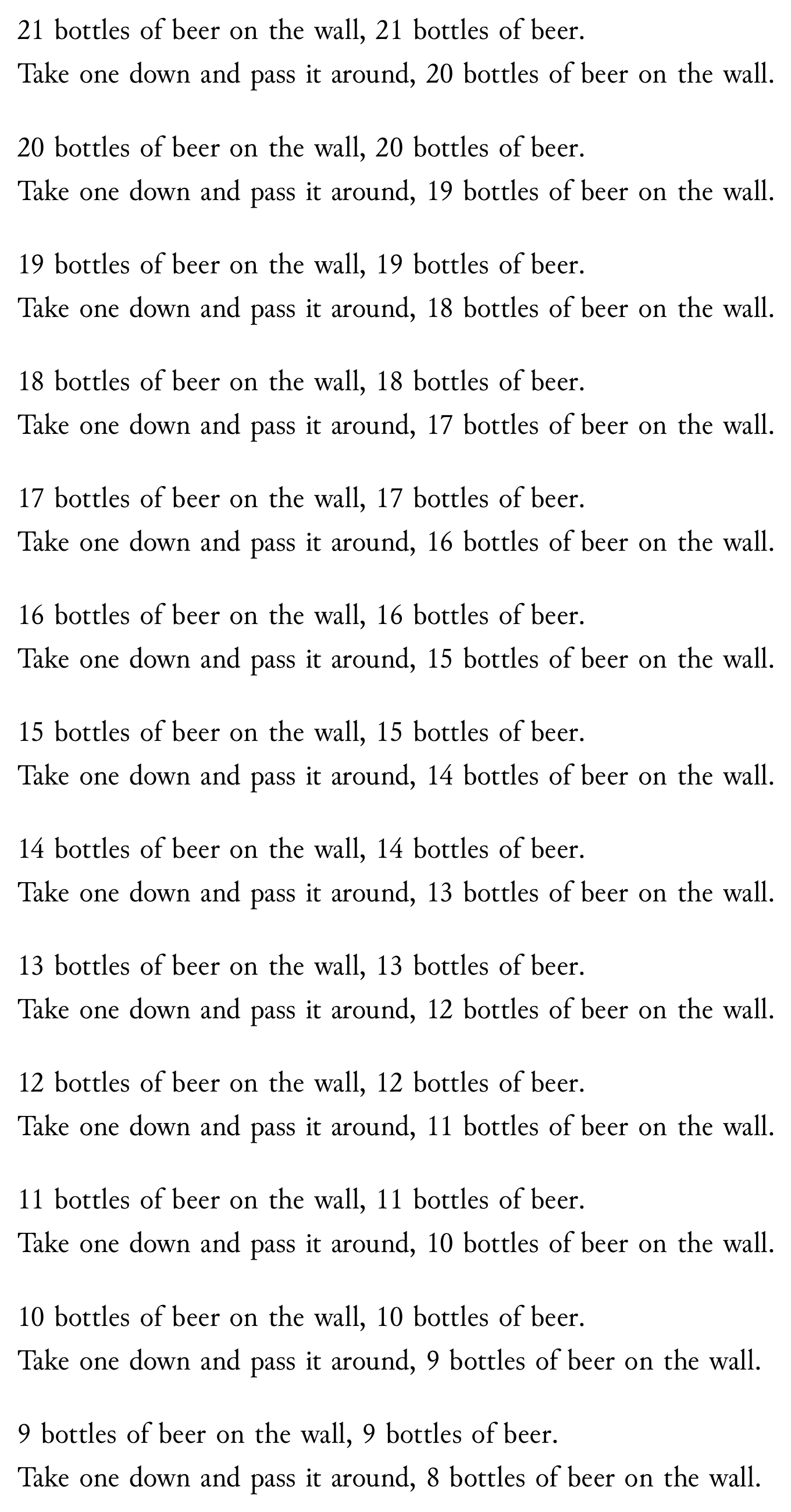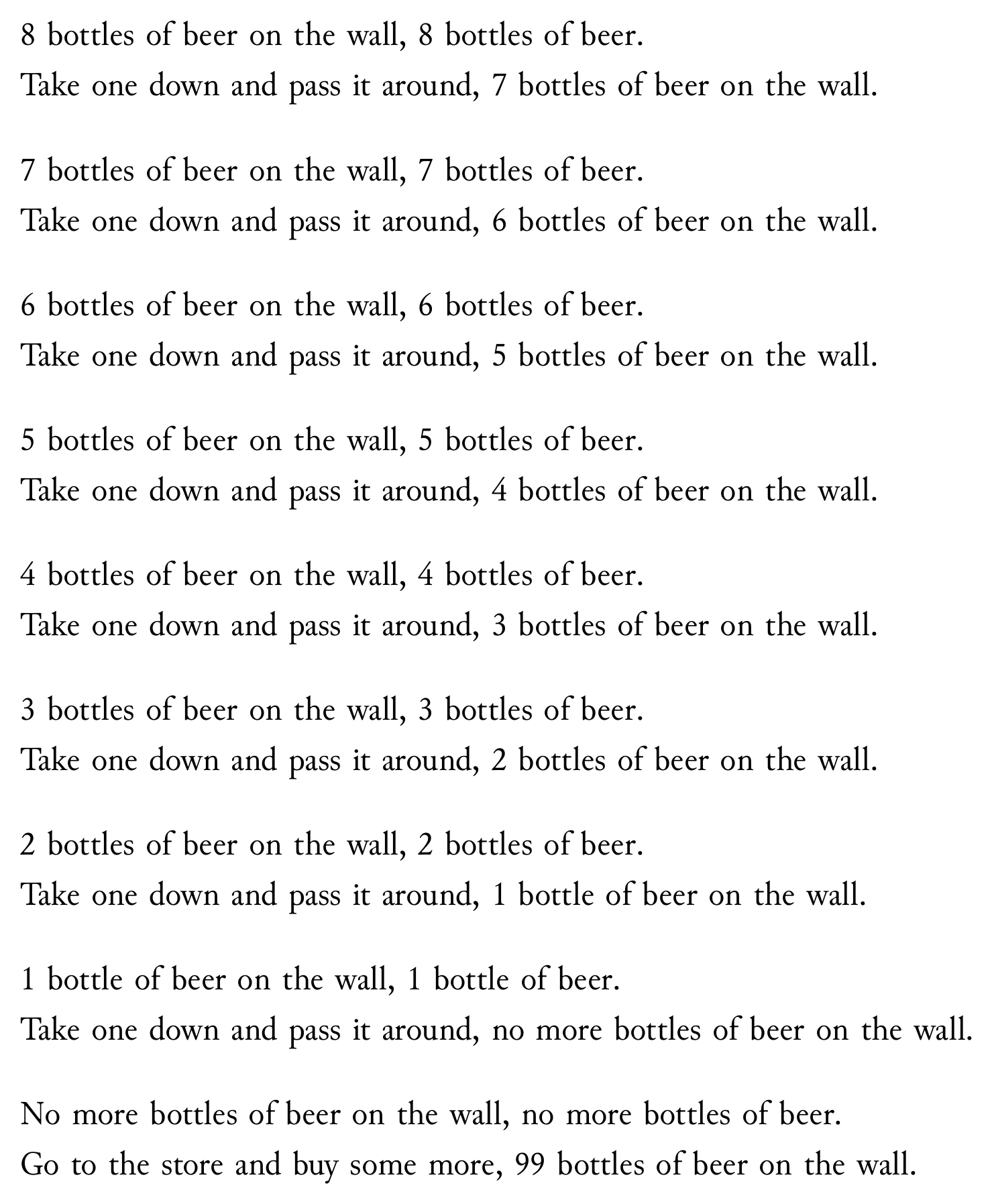# 99 Bottles of Beer

SATySFi による 99 Bottles of Beer です。

コード例

``````@require: pervasives
@require: list
@require: stdja
@require: standalone

let-rec range n =
if n <= 0 then []
else (n - 1) :: (range (n - 1))

let newline =
discretionary (-10000) inline-nil inline-nil inline-nil

let bottles-of-beer n =
let n-str = arabic n in
if n <= 0 then
`No more bottles of beer on the wall, no more bottles of beer.`
else if n == 1 then
`1 bottle of beer on the wall, 1 bottle of beer.`
else
n-str ^ #` bottles of beer on the wall, `# ^ n-str ^ #` bottles of beer.`

let take-one n =
let prefix = `Take one down and pass it around, `# in
if n <= -1 then
`Go to the store and buy some more, 99 bottles of beer on the wall.`
else if n == 0 then
prefix ^ `no more bottles of beer on the wall.`
else if n == 1 then
prefix ^ `1 bottle of beer on the wall.`
else
prefix ^ (arabic n) ^ #` bottles of beer on the wall.`

let-block ctx +ninety-nine-bottles-of-beer =
let aux n =
let ib-of-line s = (s |> embed-string |> read-inline ctx) ++ inline-fil in
let first = ib-of-line (bottles-of-beer n) in
let second = ib-of-line (take-one (n - 1)) in
let ib = first ++ newline ++ second in
form-paragraph ctx ib
in
(range 100)
|> List.map aux
|> List.fold-left (+++) block-nil

in
standalone '<
+ninety-nine-bottles-of-beer;
>
``````

コード例の組版結果 (SATySFi version 0.0.3)